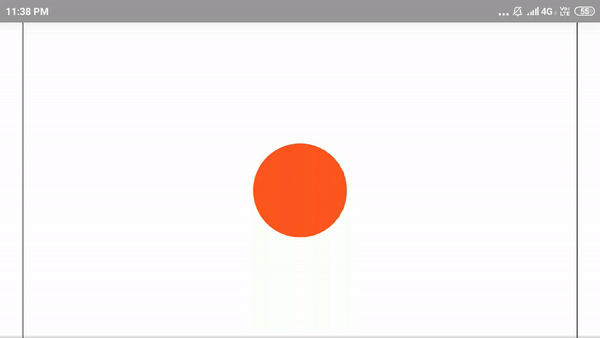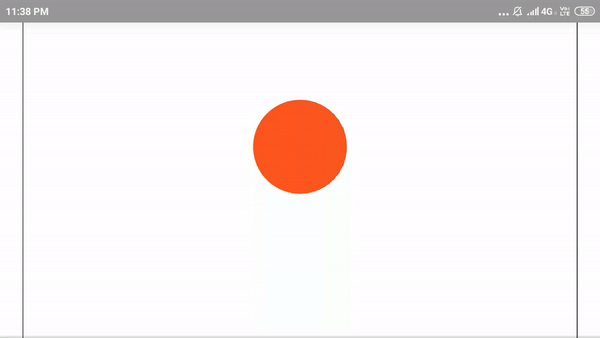# How to make smooth bounce animation using CSS ?

• Last Updated : 28 Nov, 2019

The smooth bounce animation can be done with the help of HTML and CSS. It will generate fun and desired outputs.

For this project, a simple div with class ball is needed in HTML page:

Attention reader! Don’t stop learning now. Get hold of all the important HTML concepts with the Web Design for Beginners | HTML  course.

 `
`

We will switch to CSS for animation programming. Now, Flexbox is used to have the ball at the middle of the page and make the ball 70px by 70px in size. It can be taken in any size of the user’s choice as it determines the size of the ball.

 `body {``  ``display``: flex;              ``  ``justify-``content``: ``center``;   ``}``.ball {``  ``width``: ``100px``;``  ``height``: ``100px``;``  ``border-radius: ``50%``;         ``  ``background-color``: ``#FF5722``;  ``}`

Here,
`justify-content: center` is used to center the ball horizontally.
`border-radius : 50%` turns the square into a circle.
`background-color: #FF5722` turns the circle to orange colour. There are various code notations for colors to be remembered.

Keyframe creating: Keyframes in CSS animations gives complete control over the animation. Simple use of the keyword @keyframes followed by the name of the animation, i.e, smooth bounce ball:

 `@keyframes smoothbounceball{``    ``statements``}`

Within the keyframe, use keywords from and to to make a start and end point for the animation.

 `@keyframes smoothbounceball{``    ``from {``/*starting*/``}``    ``to {``/*ending*/``}``}`

To our understanding, we can add starting and ending values to the animation. To create a bouncing effect, we need to transform the location of the ball. transform allows to modify co-ordinates of a given element. Hence the final keyframe:

 `@keyframes smoothbounceball{``    ``from { transform: translate``3``d(``0``, ``0``, ``0``);}``    ``to { transform: translate``3``d(``0``, ``200px``, ``0``);}``}`

Here, translate3d functions takes three inputs, the change in 3-dimensional axis (x, y, z). It will translate the ball in 3-Dimensional axes. If the ball wants to move up and down, the ball needs to translate along the y-axis.

Running the keyframe: Since the @keyframe has been created, now it needs to run. In the above mentioned code of .ball{ }, a following a line has to be added:

 `.ball{``    ``Given statements...`` ` `    ``animation: bounce ``0.5``s;``    ``animation-``direction``: alternate;``    ``animation-iteration-count: infinite;``}`

The understanding of the animation: The animation tells ball element to use the given keyframe rule bounce and sets the length of the animation of 0.5 seconds. Then at finishing, the animation direction alternates. Then runs the animation an infinite number of times.

But it does not like the ball bounces but moves back and forth, up and down.
Hence, it looks like:That is because the timing of the animation is off. Animations are set to ease, by default. So, to look like the ball is bouncing, the animation needs to be slow at the start and speeding up in the middle and then finishing slowly.

Hence bezier curve is used to customize animation timings. Therefore the code:

 `.ball{``    ``Given statements..`` ` `    ``animation: bounce ``0.5``s cubic-bezier(``0.5``, ``0.05``, ``1``, ``0.5``);``}`

After this, the ball shows the bouncing effect. Here is the final code:

 ```<``html``>`` ` `<``head``>``    ``<``style``>``        ``body {``            ``display: flex;``            ``justify-content: center;``        ``}``         ` `        ``.ball {``            ``width: 100px;``            ``height: 100px;``            ``border-radius: 50%;``            ``background-color: #FF5722;``            ``animation: bounce 0.5s;``            ``animation-direction: alternate;``            ``animation-timing-function: cubic-bezier(.5, 0.05, 1, .5);``            ``animation-iteration-count: infinite;``        ``}``         ` `        ``@keyframes bounce {``            ``from {``                ``transform: translate3d(0, 0, 0);``            ``}``            ``to {``                ``transform: translate3d(0, 200px, 0);``            ``}``        ``}``        ``/* Prefix Support */``         ` `        ``ball {``            ``-webkit-animation-name: bounce;``            ``-webkit-animation-duration: 0.5s;``            ``-webkit-animation-direction: alternate;``            ``-webkit-animation-timing-function: cubic-bezier(``            ``.5, 0.05, 1, .5);``            ``-webkit-animation-iteration-count: infinite;``        ``}``         ` `        ``@-webkit-keyframes bounce {``            ``from {``                ``-webkit-transform: translate3d(0, 0, 0);``                ``transform: translate3d(0, 0, 0);``            ``}``            ``to {``                ``-webkit-transform: translate3d(0, 200px, 0);``                ``transform: translate3d(0, 200px, 0);``            ``}``        ``}``    `````` ` `<``body``>``    ``<``div` `class``=``"ball"``>```` ` ``

Output:My Personal Notes arrow_drop_up